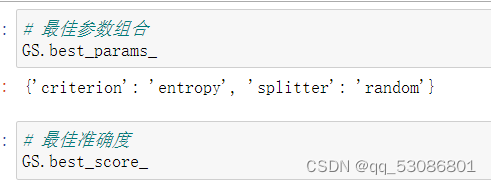# 1.概述

*子节点和父节点：在两个相连的节点中，更接近根节点的是父节点，另一个是子节点。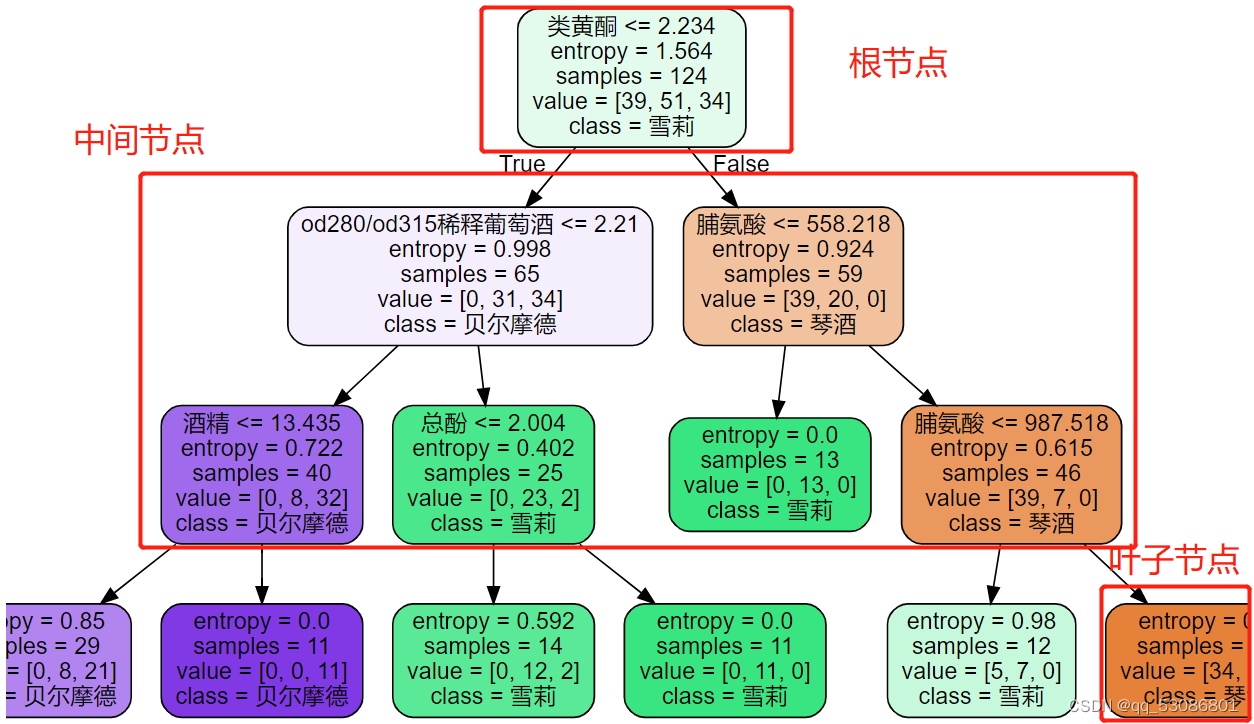# 2.sklearn中的决策树

sklearn中决策树的类都在”tree“这个模块之下。这个模块总共包含五个类：

tree.DecisionTreeClassifier分类树
tree.DecisionTreeRegressor回归树
tree.export_graphviz将生成的决策树导出为DOT格式，画图专用
tree.ExtraTreeClassifier高随机版本的分类树
tree.ExtraTreeRegressor高随机版本的回归树

sklearn建模的基本流程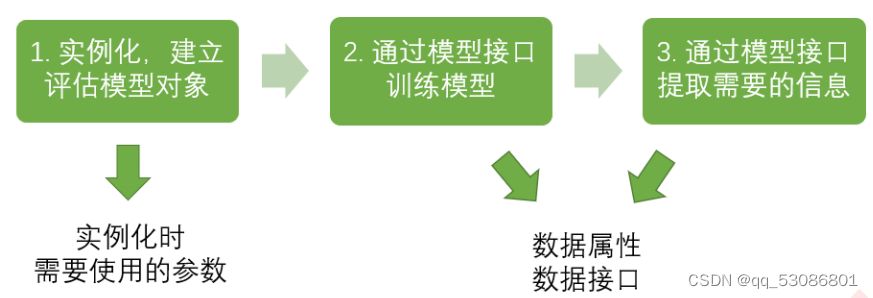``````from sklearn import tree #导入需要的模块
clf = tree.DecisionTreeClassifier()     #实例化
clf = clf.fit(X_train,y_train) #用训练集数据训练模型
result = clf.score(X_test,y_test) #导入测试集，从接口中调用需要的信息
``````

## 2.1分类树DecisionTreeClassifier

``````sklearn.tree.DecisionTreeClassifier (criterion=’gini’
, splitter=’best’
,max_depth=None
,min_samples_split=2
, min_samples_leaf=1
, min_weight_fraction_leaf=0.0
, max_features=None
,random_state=None
, max_leaf_nodes=None
, min_impurity_decrease=0.0
, min_impurity_split=None
,class_weight=None
, presort=False
)
``````

### 2.1.1 重要参数

#### （1）criterion

Criterion参数决定不纯度的计算方法。sklearn提供了两种选择：
1）输入”entropy“，使用信息熵（Entropy）
2）输入”gini“，使用基尼系数（Gini Impurity）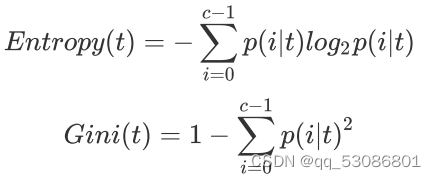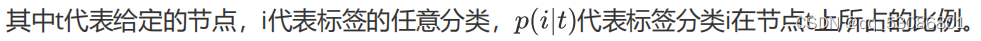1. 导入需要的算法库和模块
``````from sklearn import tree #导入模块
from sklearn.model_selection import train_test_split
``````
1. 查看数据
``````wine.data#特征值
``````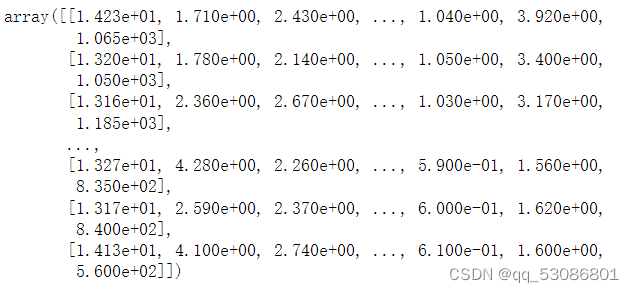``````wine.target#标签值
``````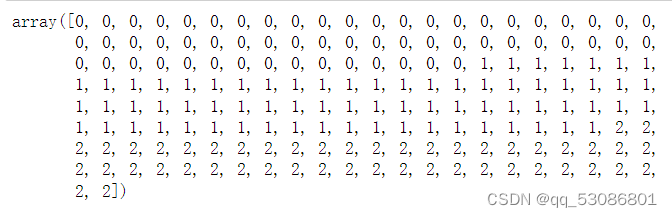``````import pandas as pd
pd.concat([pd.DataFrame(wine.data),pd.DataFrame(wine.target)],axis=1)
``````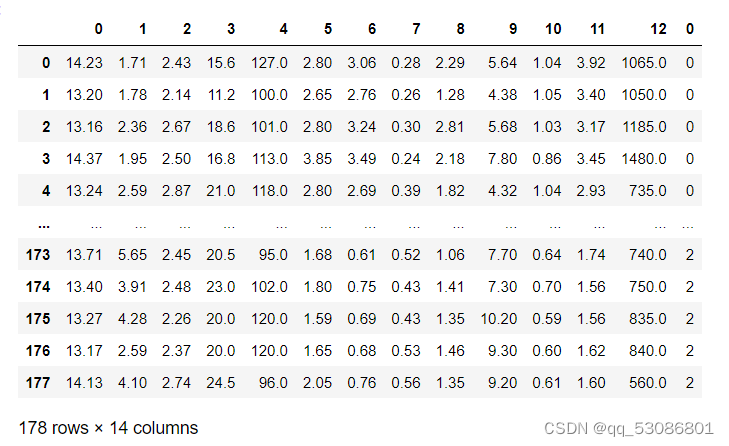1. 划分数据集为训练集和测试集
``````#划分数据集
x_train,x_test,y_train,y_test = train_test_split(wine.data,wine.target,test_size=0.3)
``````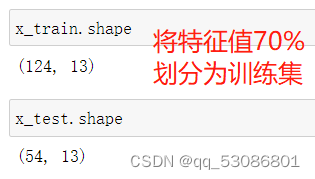1. 建立模型
``````#实例化模型
clf = tree.DecisionTreeClassifier(criterion='entropy')
#使用fit方法训练模型
clf = clf.fit(x_train,y_train)
#模型评估
score = clf.score(x_test,y_test)
``````
1. 画决策树
``````import graphviz
feature_name = ['酒精','苹果酸','灰','灰的碱性','镁','总酚','类黄酮','非黄烷类酚类','花青素','颜色强度','色调','od280/od315稀释葡萄酒','脯氨酸']
dot_data = tree.export_graphviz(clf
,feature_names=feature_name
,class_names=["琴酒","雪莉","贝尔摩德"]
,filled=True
,rounded=True

)#filled是否填充颜色， rounded框是否圆角

graph = graphviz.Source(dot_data)
graph
``````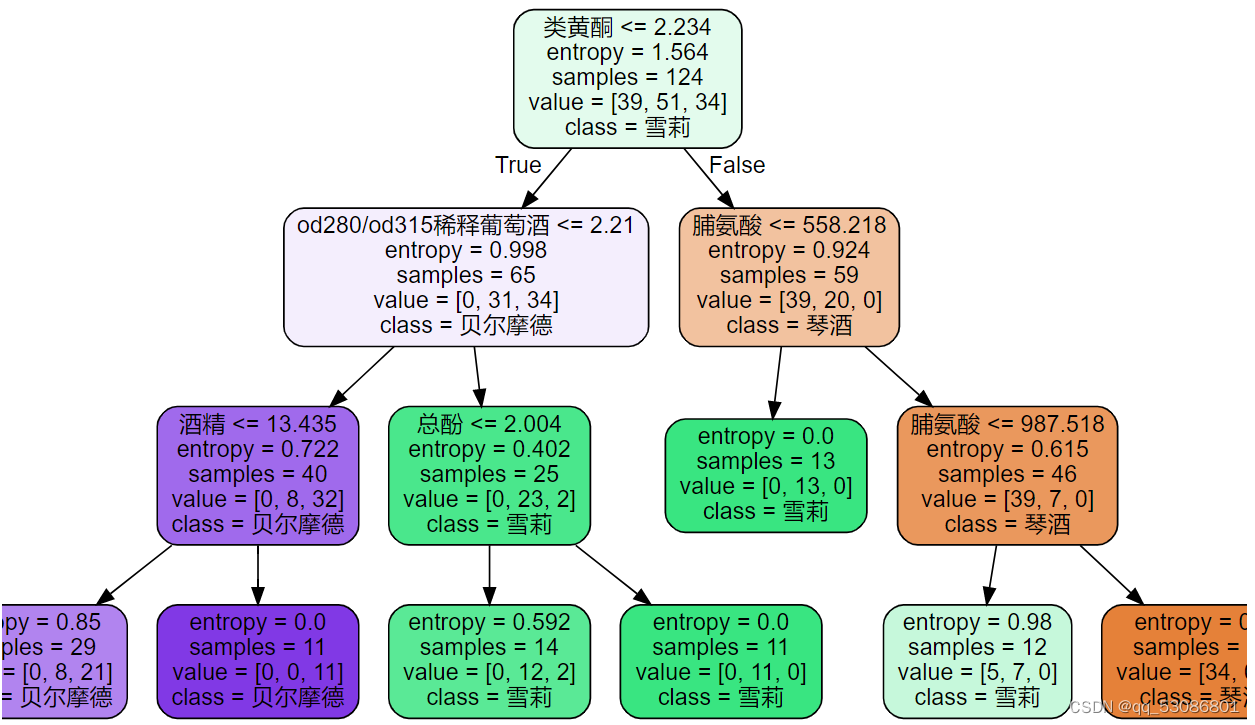1. 查看特征重要性
``````# 不同特征重要性
clf.feature_importances_
``````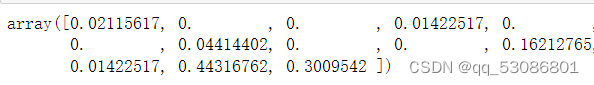``````# 特征名字和对应重要性
[*zip(feature_name,clf.feature_importances_)]
``````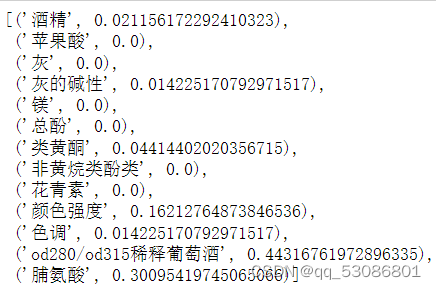sklearn在每次分枝时不使用全部特征，而是随机选取一部分特征，从中选取不纯度相关指标最优的作为分枝用的节点，因此每次生成的树也不同。

#### （2）random_state

random_state用来设置分枝中的随机模式的参数，默认None，在高维度时随机性会表现更明显，低维度的数据（比如鸢尾花数据集），随机性几乎不会显现。输入任意整数，会一直长出同一棵树，让模型稳定下来。

``````# random_state随机数种子控制决策树随机度

#实例化模型
clf = tree.DecisionTreeClassifier(criterion='entropy'
,random_state=15)#模型本身也是随机的
#使用fit方法训练模型
clf = clf.fit(x_train,y_train)
#模型评估
score = clf.score(x_test,y_test)
score
``````

#### （3）splitter

splitter也是用来控制决策树中的随机选项的。

``````clf = tree.DecisionTreeClassifier(criterion='entropy'
,random_state=20
,splitter='random'
)
clf = clf.fit(x_train, y_train)
score = clf.score(x_test, y_test)
score
``````

### 2.1.2 剪枝参数（防止过拟合）

``````# 训练集准确度
score_train = clf.score(x_train, y_train)
score_train
``````
``````# 测试集准确度
score_test = clf.score(x_test, y_test)
score_test
``````

#### （2）min_samples_leaf

min_samples_leaf限定，一个节点在分枝后的每个子节点都必须包含至少min_samples_leaf个训练样本，否则分枝就不会发生，或者，分枝会朝着满足每个子节点都包含min_samples_leaf个样本的方向去发生

#### （3）min_samples_split

min_samples_split限定，一个节点必须要包含至少min_samples_split个训练样本，这个节点才允许被分枝，否则分枝就不会发生。

``````# max_depth 限制树的最大深度，超过设定深度的树枝全部剪掉
clf = tree.DecisionTreeClassifier(criterion="entropy"
,random_state=30
,splitter="random"
,max_depth=3
,min_samples_leaf=10
,min_samples_split=10
)
clf = clf.fit(x_train, y_train)
dot_data = tree.export_graphviz(clf
,feature_names= feature_name
,class_names=["琴酒","雪莉","贝尔摩德"]
,filled=True
,rounded=True)
graph = graphviz.Source(dot_data)
graph
``````

#### （4）max_features

max_features限制分枝时考虑的特征个数，超过限制个数的特征都会被舍弃。和max_depth异曲同工，max_features是用来限制高维度数据的过拟合的剪枝参数，但其方法比较暴力，是直接限制可以使用的特征数量而强行使决策树停下的参数，在不知道决策树中的各个特征的重要性的情况下，强行设定这个参数可能会导致模型学习不足。如果希望通过降维的方式防止过拟合，建议使用PCA，ICA或者特征选择模块中的降维算法。

#### （5）min_impurity_decrease

min_impurity_decrease限制信息增益的大小，信息增益小于设定数值的分枝不会发生。

``````# 超参数的学习曲线
import matplotlib.pyplot as plt
test = []
for i in range(10):
clf = tree.DecisionTreeClassifier(max_depth=i+1
,criterion="entropy"
,random_state=30
,splitter="random"
)
clf = clf.fit(x_train, y_train)
score = clf.score(x_test, y_test)
test.append(score)
plt.plot(range(1,11),test,color="red",label="max_depth")
plt.legend()
plt.show()
``````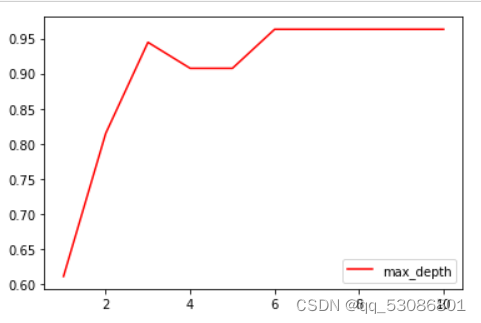### 2.1.3 目标权重参数

class_weight & min_weight_fraction_leaf

### 2.1.4 重要属性和接口

sklearn中许多算法的接口都是相似的，比如说我们之前已经用到的fit和score，几乎对每个算法都可以使用。除了这两个接口之外，决策树最常用的接口还有apply和predict。

apply中输入测试集返回每个测试样本所在的叶子节点的索引。
predict输入测试集返回每个测试样本的标签。

``````#apply返回每个测试样本所在的叶子节点的索引
clf.apply(x_test)
#predict返回每个测试样本的分类/回归结果
clf.predict(x_test)
``````

## 2.2 回归树 DecisionTreeRegressor

``````sklearn.tree.DecisionTreeRegressor (criterion=’mse’
, splitter=’best’
, max_depth=None
,min_samples_split=2
, min_samples_leaf=1
, min_weight_fraction_leaf=0.0
, max_features=None
,random_state=None
, max_leaf_nodes=None
, min_impurity_decrease=0.0
, min_impurity_split=None
, presort=False
)
``````

### 2.2.1重要参数，属性及接口

criterion

1）输入"mse"使用均方误差mean squared error(MSE)，父节点和叶子节点之间的均方误差的差额将被用来作为特征选择的标准，这种方法通过使用叶子节点的均值来最小化L2损失
2）输入“friedman_mse”使用费尔德曼均方误差，这种指标使用弗里德曼针对潜在分枝中的问题改进后的均方误差
3）输入"mae"使用绝对平均误差MAE（mean absolute error），这种指标使用叶节点的中值来最小化L1损失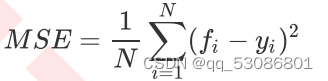``````MSE的本质，是样本真实数据与回归结果的差异。

``````

R平方被定义如下：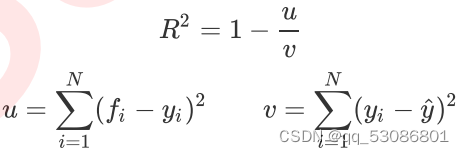R平方可以为正为负（如果模型的残差平方和远远大于模型的总平方和，模型非常糟糕，R平方就会为负），而均方误差永远为正。

``````from sklearn.datasets import load_boston
from sklearn.model_selection import cross_val_score
from sklearn.tree import DecisionTreeRegressor

regressor = DecisionTreeRegressor(random_state=0)
cross_val_score(regressor       #实例化的模型
, boston.data   #完整的特征值
, boston.target #完整的目标值
, cv=10         #几折交叉验证
,scoring = "neg_mean_squared_error"   #对于回归，默认返回R²。在回归交叉验证要使用MSE
)
``````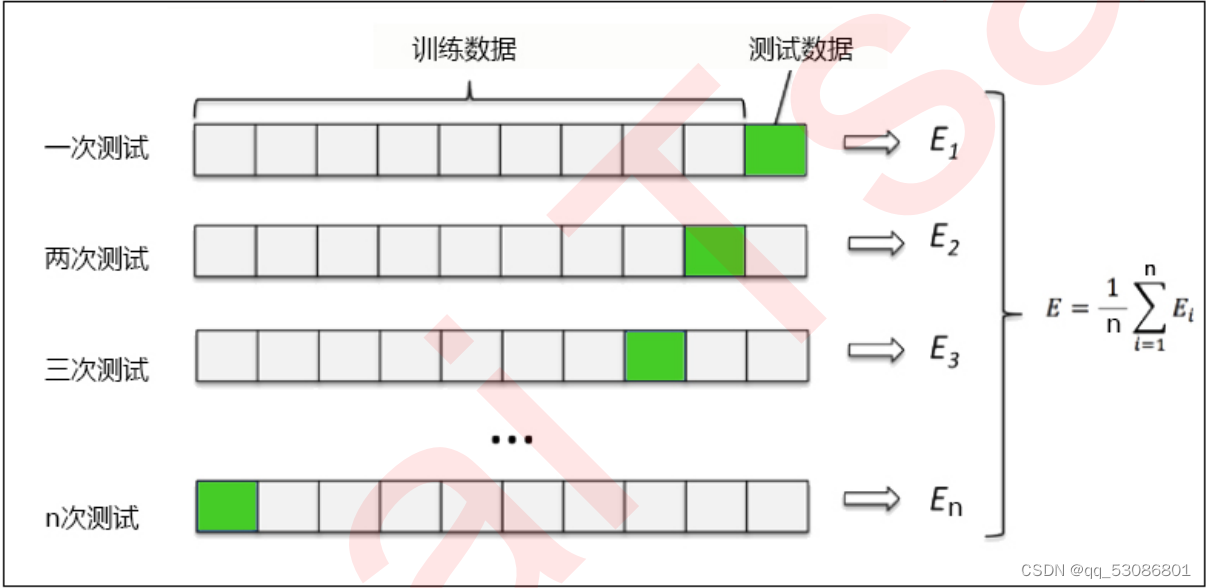### 例：一维回归的图像绘制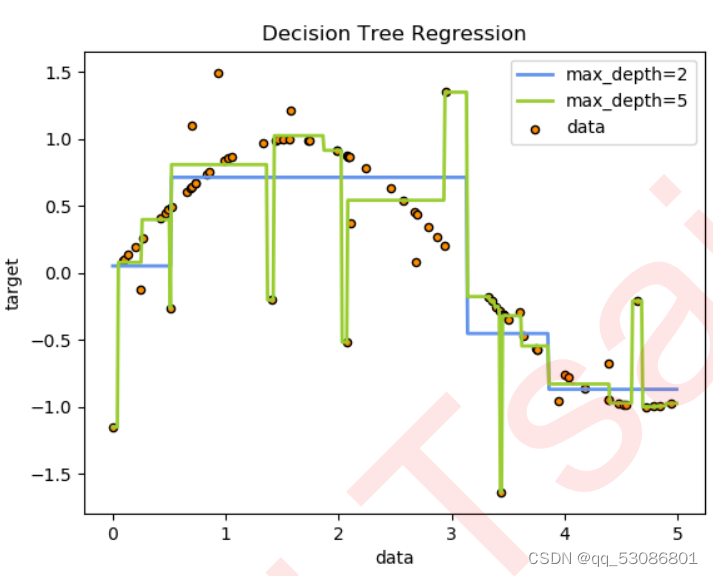``````# 1. 导入需要的库
import numpy as np
from sklearn.tree import DecisionTreeRegressor
import matplotlib.pyplot as plt
``````
``````# 2. 创建一条含有噪声的正弦曲线
rng = np.random.RandomState(1)  #随机数种子
X = np.sort(5 * rng.rand(80,1), axis=0) # rng.rand(80,1)生成一个二维数组，np.sort排序
y = np.sin(X).ravel()
y[::5] += 3 * (0.5 - rng.rand(16)) #加噪声数据
#np.random.rand(数组结构)，生成随机数组的函数
``````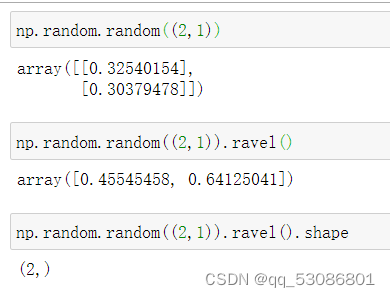``````# 3. 实例化&训练模型
regr_1 = DecisionTreeRegressor(max_depth=2)
regr_2 = DecisionTreeRegressor(max_depth=5)
regr_1.fit(X, y)
regr_2.fit(X, y)
``````
``````# 4. 测试集导入模型，预测结果
X_test = np.arange(0.0, 5.0, 0.01)[:, np.newaxis]
y_1 = regr_1.predict(X_test)
y_2 = regr_2.predict(X_test)
#np.arrange(开始点，结束点，步长) 生成有序数组的函数
``````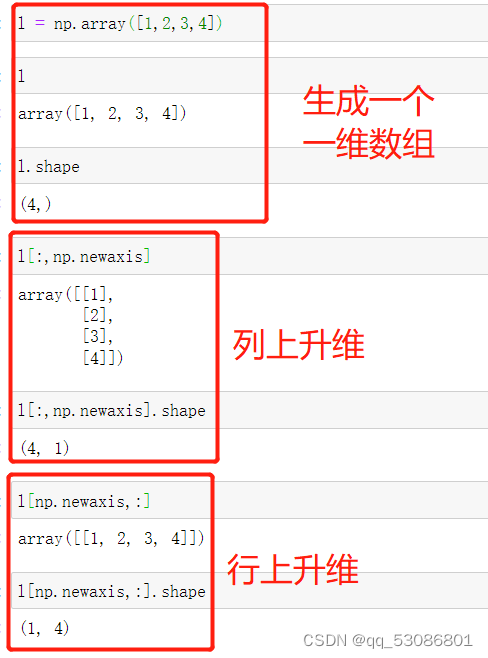``````# 绘制图像
plt.figure()
plt.scatter(X, y, s=20, edgecolor="black",c="darkorange", label="data")
plt.plot(X_test, y_1, color="cornflowerblue",label="max_depth=2", linewidth=2)
plt.plot(X_test, y_2, color="yellowgreen", label="max_depth=5", linewidth=2)
plt.xlabel("data")
plt.ylabel("target")
plt.title("Decision Tree Regression")
plt.legend()
plt.show()
``````

# 实例：泰坦尼克号幸存者的预测

1. 导入所需要的库
``````import pandas as pd
from sklearn.tree import DecisionTreeClassifier
from sklearn.model_selection import train_test_split
from sklearn.model_selection import GridSearchCV
from sklearn.model_selection import cross_val_score
import matplotlib.pyplot as plt
``````
1. 导入数据集，探索数据
``````data = pd.read_csv("F:/jupyter notebook/data.csv")
``````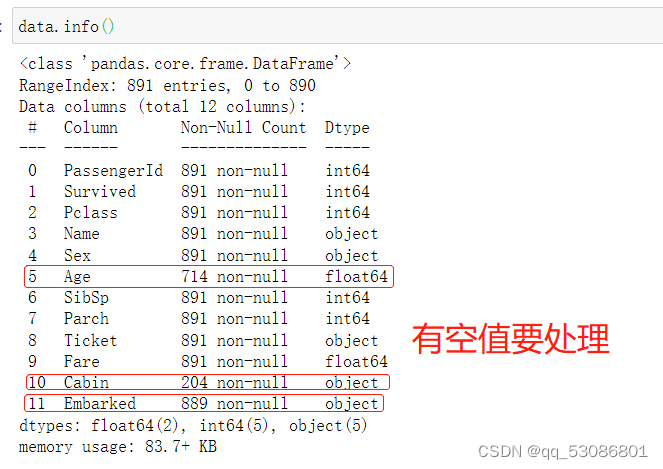1. 对数据集进行预处理
``````#删除缺失值过多的列，和观察判断来说和预测的y没有关系的列
data.drop(["Cabin","Name","Ticket"],inplace=True,axis=1) #处理缺失值，对缺失值较多的列进行填补，有一些特征只确实一两个值，可以采取直接删除记录的方法
data["Age"] = data["Age"].fillna(data["Age"].mean())
data = data.dropna()
``````

``````#将二分类变量转换为数值型变量
#astype能够将一个pandas对象转换为某种类型，和apply(int(x))不同，astype可以将文本类转换为数字，用这个方式可以很便捷地将二分类特征转换为0~1
data["Sex"] = (data["Sex"]== "male").astype("int")

#将三分类变量转换为数值型变量
labels = data["Embarked"].unique().tolist()
data["Embarked"] = data["Embarked"].apply(lambda x: labels.index(x))
``````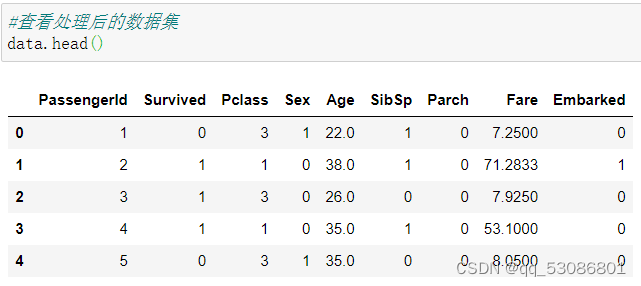1. 提取标签和特征矩阵，分测试集和训练集
``````X = data.iloc[:,data.columns != "Survived"]
y = data.iloc[:,data.columns == "Survived"]
from sklearn.model_selection import train_test_split
Xtrain, Xtest, Ytrain, Ytest = train_test_split(X,y,test_size=0.3)

#修正测试集和训练集的索引
for i in [Xtrain, Xtest, Ytrain, Ytest]:
i.index = range(i.shape)
``````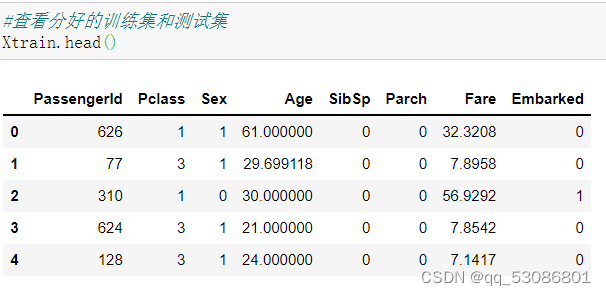1. 导入模型，粗略跑一下查看结果
``````clf = DecisionTreeClassifier(random_state=25)
clf = clf.fit(Xtrain, Ytrain)
``````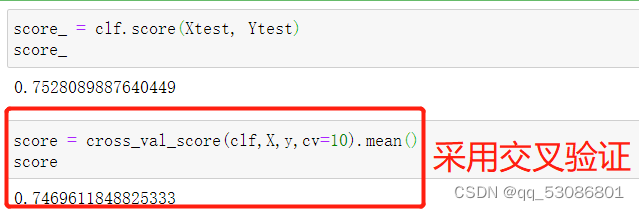1. 在不同max_depth下观察模型的拟合状况
``````tr = []
te = []
for i in range(10):
clf = DecisionTreeClassifier(random_state=25
,max_depth=i+1
,criterion="entropy"
)
clf = clf.fit(Xtrain, Ytrain)
score_tr = clf.score(Xtrain,Ytrain)
score_te = cross_val_score(clf,X,y,cv=10).mean()
tr.append(score_tr)
te.append(score_te)
print(max(te))
plt.plot(range(1,11),tr,color="red",label="train")
plt.plot(range(1,11),te,color="blue",label="test")
plt.xticks(range(1,11))
plt.legend()
plt.show()
``````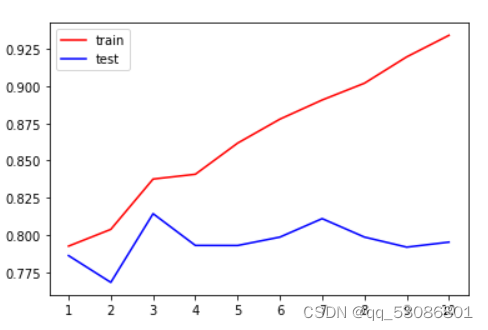1. 用网格搜索调整参数
``````import numpy as np
gini_thresholds = np.linspace(0,0.5,20)
parameters = {'splitter':('best','random')
,'criterion':("gini","entropy")
}
clf = DecisionTreeClassifier(random_state=25)
GS = GridSearchCV(clf, parameters, cv=10)
GS.fit(Xtrain,Ytrain)
``````